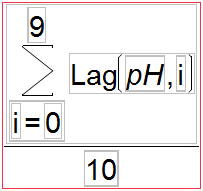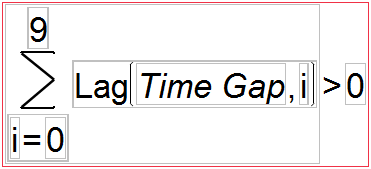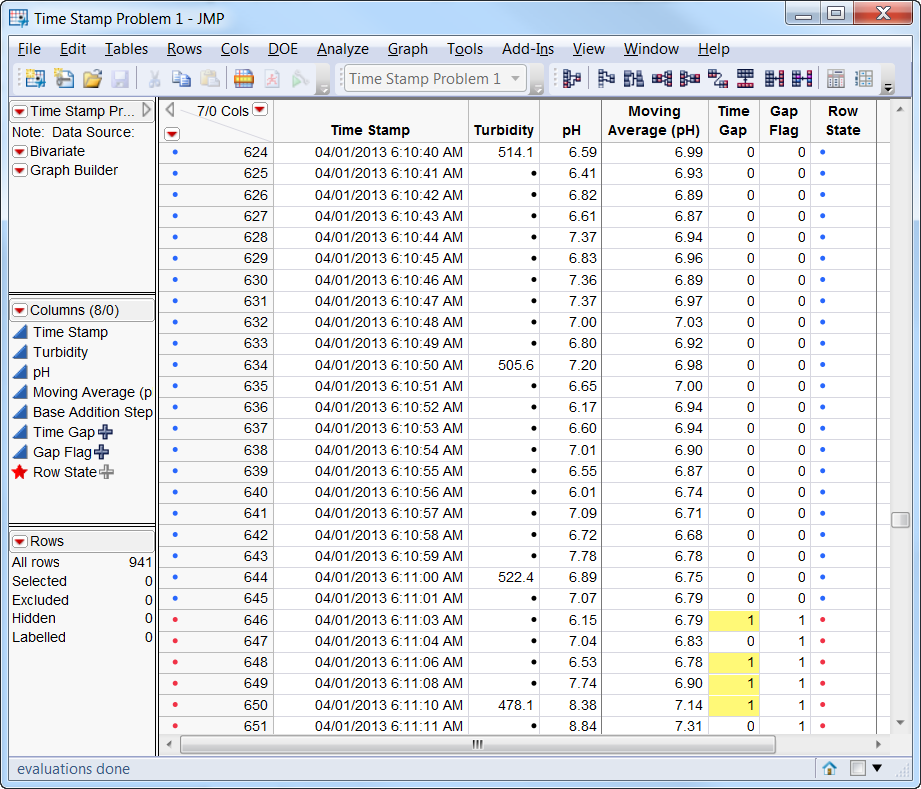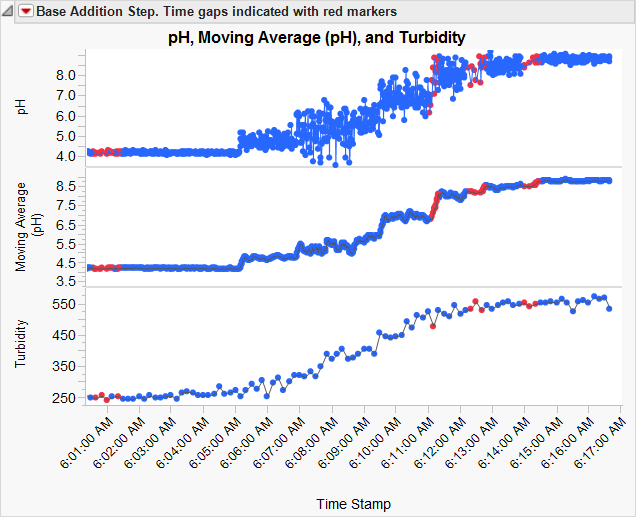World Statistics Day was yesterday, but we’re celebrating all week long! This celebration means acknowledging the impact statistics has on our world. Who is your favorite statistician? Share with us who they are and why they top your favorites list.
Choose Language Hide Translation Bar
Easy moving average using Summation function

Scientists and engineers use on-line sensors and measurement devices to monitor an almost infinite array of process attributes. When analyzing time series data captured by historians and data loggers, two questions come up regularly: “How can I calculate a moving average?” and a related question: “How do I flag rows after a gap occurs in my time series data?”

JMP can do both easily, but the answer requires a little knowledge of a less frequently used  column formula function.

It is often convenient to calculate a moving average for time-sampled data. This both dampens the noise in the data stream and lets us have an average measurement value at a specific point in time. For example, in the data table in Figure 4, I have an on-line pH meter that is recording a measurement once every second, but my turbidity meter can only record a measurement every 10 seconds. I could just match the 10-second turbidity reading with the corresponding pH measurement, but the turbidity reading actually represents the previous 10 seconds of what the instrument saw, and I would like to compare it to a similar 10-second time resolution of pH. To do this, I will need to calculate the moving average of the past 10 seconds of pH measurements. The 1-second resolution and 10-second moving averages are compared in the Graph Builder plot below the table in Figure 5.

The second question comes up when we are using moving averages. One assumption we have with a moving average is that each measurement is equally spaced. This makes the moving average uniformly weighted over all intervals. But periodically (in real life), there are short gaps in the data stream, and gaps longer than some meaningful length of time need to be identified to alert us that the assumption has broken down. These gaps are caused by a variety of issues, like short circuits, intermittent drop outs or just missing data from network connected devices.

A moving average can be calculated in a single step using the Summation function in the column formula editor.As text, the formula in Figure 1 is Summation( i = 0, 9, Lag( :pH, i ) ) / 10

The summation function (Figure 1) works like an iterative loop. In this case, while ”i” is between 0 and 9, it calculates the sum of the values it gets from the argument at the right. The argument on the right is evaluated as Lag(:pH,0), Lag(:pH,1), Lag(:pH,2), Lag(:pH,3)… Lag(:pH,9). In this case, Lag(:pH,0) is the value at the current row of the pH column, and Lag(:pH,9) is the value 9 rows above the current row (for a total of 10 rows).

The second problem is a little tricky, and it will be easier to break it down into two steps. In step one, we will make a formula column to flag the large time gaps, and then in step two, we will add a second formula that will flag a range of rows after the time gap.

The first step is to make a new column named “Time Gap” and then add a formula to flag gaps when they are longer than 5 seconds like so:

:Time Stamp - Lag( :Time Stamp, 1 ) > 5

Or if you wanted to step it up a little you could use the Date Difference function like this:

Date Difference( Lag( :Time Stamp, 1 ), :Time Stamp, "second" ) > 5

In this specific case, I wanted to know when time gaps of 5 seconds or longer happened; in other projects, I really only needed to know when longer gaps occurred.

The Date difference function calculates the difference between two date values and returns the result in units that can be specified (e.g., hour, minute, day, week, year). In both cases, if the difference is greater than 30, then the formula is evaluated as “1” for true and “0” for false.

In the second step, we make another new column name “Gap Flag” and add a formula to mark 10 rows following the gap detected in the time gap column.As text the formula in Figure 2 is Summation( i = 0, 9, Lag( :Time Gap, i ) ) > 0

Again, the Summation function (Figure 2) works like an iterative loop. This time, it is combined with a conditional argument. While ”i” is between 0 and 9, if the sum of the values in the Time Gap column is greater than 0, then the formula evaluated as “1” for true or “0” for false. If there is at least one “1” in the Gap Time Column for the previous 30 rows, then the value in this flag column is 1 also.

With just a couple of little tweaks, we can have this formula set the color of the row maker, too. To use this formula, make a new column and set the data type to “Row State.”

If( Summation( i = 0, 9, Lag( :Time Gap, i ) ) > 0,

Row State() = Color State( "red" ),

Row State() = Color State( "blue" )

);

The Summation function is an important tool in your JMP toolbox, and it can save a lot of time and effort. For more information on syntax and use of the Summation function, check out Using JMP, the Scripting Guide books and the Scripting Index -- all are found under the Help menu in JMP.Article Labels

There are no labels assigned to this post.

Article Tags